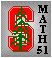## Math 51 Winter 2020

 Home Textbook Syllabus Homework Enrollment Office hours and help Exams
 The schedule of the topics is tentative and will be adjusted as necessary.
 Week 1 (1/6-1/10) [1/6 Preliminary study list deadline] [1/6 First lecture; 1/7 first section] Chapter 1: Vectors and related algebra (addition, scalar multiplication) Chapter 2: Vector geometry (length, dot product, angle) and correlation Chapter 3: Many ways to think about planes in space (algebraic and geometric)
 Week 2 (1/13-1/17) Chapter 4: Span, subspace, and dimension Chapter 5: Basis and orthogonality Chapter 6: Projection onto subspaces Chapter 7: Application of projections: linear regression
 Week 3 (1/20-1/24) [1/24 Final study list deadline] Chapter 8: Multivariable functions, level sets, and contour plots Chapter 9: Partial derivatives and how to visualize them
 Week 4 (1/27-1/31) [1/30 Midterm 1, approximately 7:30pm ; roughly covers through end of Week 3 topics listed above] Chapter 10: Multivariable extrema via critical points Midterm 1 Review Chapter 11: Gradient and linear approximation
 Week 5 (2/3-2/7) Chapter 12: Solving constrained optimization via Lagrange multipliers Chapter 13: Linear functions, matrices, and the derivative matrix Chapter 14: Linear transformations and matrix multiplication
 Week 6 (2/10-2/14) Chapter 15: Matrix algebra Chapter 16: Applications of matrix algebra: Markov chains and feedback loops Chapter 17: Multivariable Chain Rule
 Week 7 (2/17-2/21) [2/20 Midterm 2, approximately 7:30pm ; covers through end of Week 6 topics listed above] Chapter 18: Matrix inverses and multivariable Newton's method Chapter 19: Linear independence and the Gram-Schmidt process
 Week 8 (2/24-2/28) [2/28, 5pm: Course withdrawal and change of grading basis deadline] Chapter 20: Matrix transpose, orthogonal matrices, and quadratic forms Chapter 21: Systems of linear equations, column space, and null space Chapter 22: Matrix decompositions (LU and QR)
 Week 9 (3/2-3/6) Chapter 23: Eigenvalues and eigenvectors Chapter 24: Applications of eigenvalues: matrix powers, Spectral Theorem, and geometry of quadratic forms Chapter 25: Second partial derivatives and Hessian matrix Chapter 26: Application of Hessian: multivariable second derivative test for local extrema
 Week 10 (3/9-3/13) [3/12 Last section; 3/13 last lecture] [3/17 Final Exam, 12:15-3:15pm; comprehensive but more heavily emphasizes topics since Midterm 2] Chapter 25 and 26, leftovers Chapter 27: More applications of eigenvalues (especially singular value decomposition) (not covered on final exam) Final Exam Review

Winter 2020 -- Department of Mathematics, Stanford University